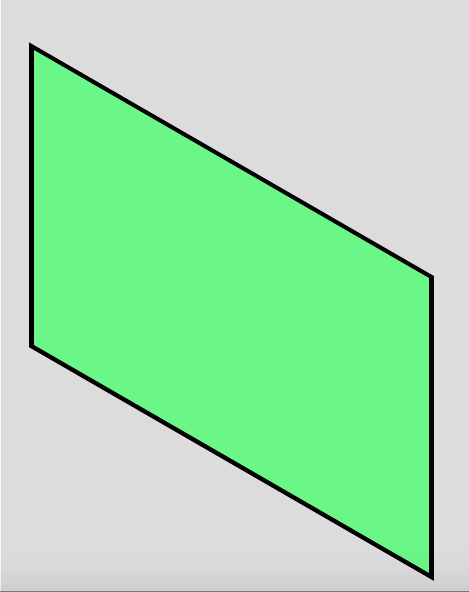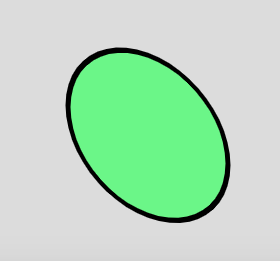GeeksforGeeks App
Open AppBrowser
Continue

# p5.js | Typography | shearY() Function

The shearY() function in p5.js is used to shear a shape or object around the y-axis. The object is sheared by the amount specified by the angle parameter. For proper functioning of angles should be specified in the current angleMode. The objects are always sheared around their relative position to the origin and in a clockwise direction.

Syntax:

`shearY(angle)`

Parameters: This function accepts single parameter angle which stores the value of angle.

Below programs illustrate the shearY() function in p5.js:

Example 1: This example uses shearY() function to shear the object around y-axis.

 `function` `setup() {`` ` `    ``// Create Canvas of given size``    ``createCanvas(780, 650);``}`` ` `function` `draw() {``     ` `    ``// Set the background color``    ``background(220);``     ` `    ``// Set fill color``    ``fill(``'lightgreen'``);``     ` `    ``// Set stroke width``    ``strokeWeight(5);``     ` `    ``// Set shearY function``    ``shearY(PI/6);``     ` `    ``// Finally Draw the sheared rectangle``    ``rect(30, 30, 400, 300);``}`

Output:Example 2: This example uses shearY() function to shear the object around y-axis.

 `function` `setup() {``     ` `    ``// Create Canvas of given size``    ``createCanvas(780, 650);``}`` ` `function` `draw() {``     ` `    ``// Set the background color``    ``background(220);``     ` `    ``// Set fill color``    ``fill(``'lightgreen'``);``     ` `    ``// Set stroke width``    ``strokeWeight(5);``     ` `    ``// Set shearY function``    ``shearY(PI/9);``     ` `    ``// Finally Draw the sheared``    ``// circle of radius 80 ``    ``circle(height/2, width/2, 80);``}`

Output:Reference: https://p5js.org/reference/#/p5/shearY

My Personal Notes arrow_drop_up
Related Tutorials